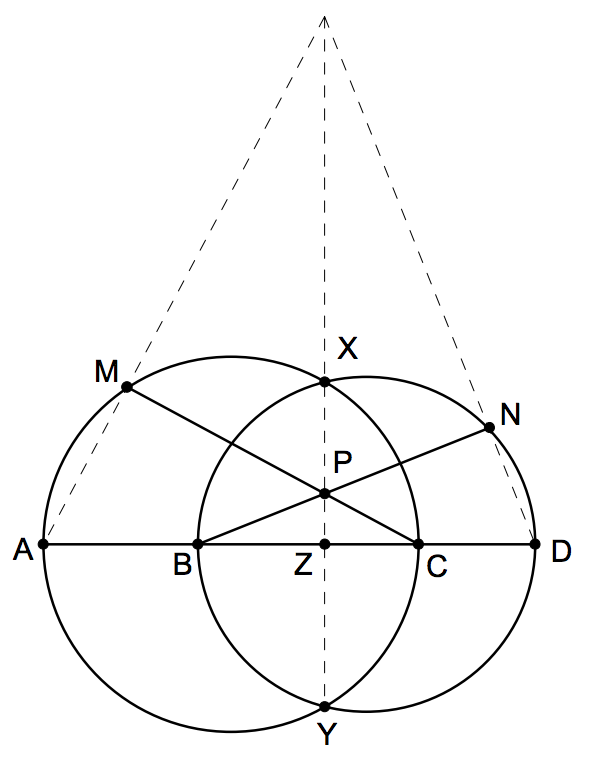###### back to index | new

Let $S = {1, 2, ..., n}$, where $n \ge 1$. Each of the $2^n$ subsets of $S$ is to be colored red or blue. (The subset itself is assigned a color and not its individual elements.) For any set $T \subseteq S$, we then write $f(T)$ for the number of subsets of T that are blue. Determine the number of colorings that satisfy the following condition: for any subsets $T_1$ and $T_2$ of $S$, $f(T_1)f(T_2) = f(T_1 \cup T_2)f(T_1 \cap T_2).$

Steve is piling $m \geq 1$ indistinguishable stones on the squares of an $n\times n$ grid. Each square can have an arbitrarily high pile of stones. After he finished piling his stones in some manner, he can then perform stone moves, defined as follows. Consider any four grid squares, which are corners of a rectangle, i.e. in positions $(i, k), (i, l), (j, k), (j, l)$ for some $1\leq i, j, k, l \leq n$, such that $i < j$ and $k < l$. A stone move consists of either removing one stone from each of $(i, k)$ and $(j, l)$ and moving them to $(i, l)$ and $(j, k)$ respectively,j or removing one stone from each of $(i, l)$ and $(j, k)$ and moving them to $(i, k)$ and $(j, l)$ respectively. Two ways of piling the stones are equivalent if they can be obtained from one another by a sequence of stone moves. How many different non-equivalent ways can Steve pile the stones on the grid?

Let $a, b, c, d, e$ be distinct positive integers such that $a^4 + b^4 = c^4 + d^4 = e^5$. Show that $ac + bd$ is a composite number.

Solve in positive integers $\frac{1}{x} + \frac{1}{y} + \frac{1}{z} = \frac{4}{5}$

Prove there exist infinite number of positive integer $a$ such that for any positive integer $n$, $n^4 + a$ is not a prime number.

Prove for any positive integer $n$, the fraction $\frac{21n+4}{14n+3}$ cannot be further simplified.

If integer d is not equal to 2,5 or 13. Prove: there must exist two different elements $a$ and $b$ in set {2, 5, 3, d} such that $ab - Let$ABCD$be a cyclic quadrilateral. Let$P, Q, R$be the feet of the perpendiculars from$D$to the lines$BC, CA$and$AB$respectively. Show that$PQ = QR$iff the bisectors of$\angle ABC$and$\angle ADC$meet on$AC$. Let$ABCD$be a convex quadrilateral for which$AC = BD$. Equilateral triangles are constructed on the sides of the quadrilateral and pointing outward. Let$O_1, O_2, O_3, O_4$be the centres of the triangles constructed on$AB, BC, CD,$and$DA$respectively. Prove that lines$O_1O_3$and$O_2O_4$are perpendicular. Let$f(n)$denote the sum of the digits of$n$. Find$f(f(f(4444^{4444})))$. Prove that$\frac{5^{125}-1}{5^{25}-1}$is composite. Find all integers$a$,$b$,$c$with$1 < a < b < c$such that the number$(a-1)(b-1)(c-1)$is a divisor of$(abc-1)$. Prove that if positive integer$a$and$b$are such that$ab+1$divides$a^2 + b^2$. then $$\frac{a^2+b^2}{ab+1}$$ is a square number. Find the maximal value of$m^2+n^2$if$m$and$n$are integers between$1$and$1981$satisfying$(n^2-mn-m^2)^2=1$. Let$A$,$B$,$C$and$D$be four distinct points on a line, in that order. The circles with diameters$AC$and$BD$intersect at the points$X$and$Y$. The line$XY$meets$BC$at the point$Z$. Let$P$be a point on the line$XY$different from$Z$. The line$CP$intersects the circle with diameter$AC$at the points$C$and$M$, and the line$BP$intersects the circle with diameter$BD$at the points$B$and$N$. Prove that the lines$AM$,$DN$and$XY$are concurrentShow that$x^n + 5x^{n-1} + 3 = 0$cannot be factorized into two non-constant polynomials with integer coefficients. Let sequence$\{a_n\}$satisfy$a_0=0, a_1=1$, and$a_n = 2a_{n-1}+a_{n-2}$. Show that$2^k\mid n$if and only if$2^k\mid a_n$. Let$\{a_n\}$be a sequence defined as$a_n=\lfloor{n\sqrt{2}}\rfloor$where$\lfloor{x}\rfloor$indicates the largest integer not exceeding$x$. Show that this sequence has infinitely many square numbers. Let sequence$g(n)$satisfy$g(1)=0, g(2)=1, g(n+2)=g(n+1)+g(n)+1$where$n\ge 1$. Show that if$n$is a prime greater than 5, then$n\mid g(n)[g(n)+1]$. Seventeen people correspond by mail with one another - each one with all the rest. In their letters only three different topics are discussed. Each pair of correspondents deals with only one of these topics. Prove that there are at least three people who write to each other about the same topic. Let$n$be an integer greater than or equal to 3. Prove that there is a set of$n$points in the plane such that the distance between any two points is irrational and each set of three points determines a non-degenerate triangle with rational area. (Weitzenbock's Inequality) Let$a, b, c$, and$S$be a triangle's three sides' lengths and its area, respectively. Show that $$a^2 + b^2 + c^2 \ge 4\sqrt{3}\cdot S$$ The diagonals$AC$and$CD$of the regular hexagon$ABCDEF$are divided by inner points$M$and$N$such that$AM:AC = CN:CE=r$. Determine$r$if$B, M,$and$N$are collinear. Prove that there is one and only one triangle whose side lengths are consecutive integers, and one of whose angles is twice as large as another. If a sequence$\{a_n\}$satisfies$a_1=1$and$a_{n+1}=\frac{1}{16}\big(1+4a_n+\sqrt{1+24a_n}\big)$, find the general term of$a_n\$.